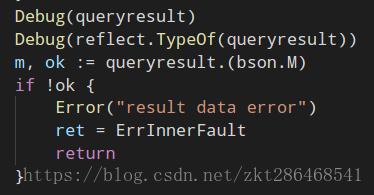Golang# 解决golang 反射interface{}做零值判断的一个重大坑

## 比如下方:

```    in := 0.
var tmp interface{} = float32(in)
fmt.Println("float 0==0:", in == 0)
fmt.Println("float -> interface{} -> float", tmp.(float32) == 0)
switch v := tmp.(type) {
case float32:
fmt.Println("float -> interface -.type-> float", v == 0)
}```

float 0==0: true

float -> interface{} -> float true

float -> interface -.type-> float true

```func f(arg interface{}){
switch v:=arg.(type) {
case float32,float64:
fmt.Println(v==0)
}
}
func main(){
f(0.)
}```

false

```//相对正确的写法
func f(arg interface{}){
switch v:=arg.(type) {
case float32,float64:
fmt.Println(v==0.)
case int,int32,in64:
fmt.Pringtln(v==0)
}
}

//错误的写法
func f(arg interface{}){
switch v:=arg.(type) {
case float32,float64,int,int64,int32:
fmt.Println(v==0)
}
}
```

```func f(arg interface{}){
switch v:=arg.(type) {
case float32,float64:
fmt.Println(v==0.)
case int,int32,in64:
fmt.Pringtln(v==0)
}
}
func main(){
var i float32
f(i)
}```

false

```func f(arg interface{}){
switch v:=arg.(type) {
case float32:
r:=float64(v)
fmt.Println(math.Abs(r-0)<0.0000001)
case float64:
fmt.Println(math.Abs(v-0)<0.0000001)
}
}```

```//编译器不通过的写法,理由是，不支持interface{}类型的v，进行float64(v)操作
func f(arg interface{}){
switch v:=arg.(type) {
case float32,float64:
r:=float64(v)
fmt.Println(math.Abs(r-0)<0.0000001)
}```

## 背景

### 1.从mongo中取数据

```newSession := m.Session.Copy()
defer newSession.Close()
c := newSession.DB(database).C(collName)
if err := c.Find(bson.M{"time": occurtime}).One(&data); err != nil {
Error(err)
}
```

### 2.mongo返回数据后 对interface数据进行解析## 问题## 解决